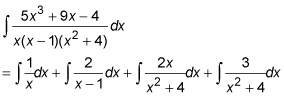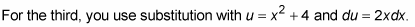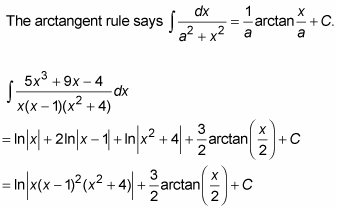##### Differential Equations For DummiesYou can use the partial fractions method to integrate rational functions, including functions with denominators that contain irreducible quadratic factors (that is, quadratic factors that can’t be broken up into linear factors).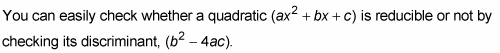If the discriminant is negative, the quadratic is irreducible.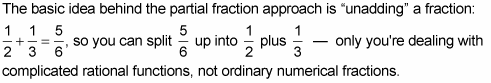You can use the partial fractions technique for functions whose denominators can be factored down to linear factors. However, using this technique is a bit different when there are irreducible quadratic factors.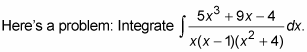1. Factor the denominator.

2. Break up the fraction into a sum of “partial fractions.”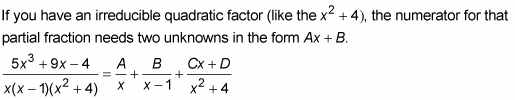3. Multiply both sides of this equation by the left-side denominator.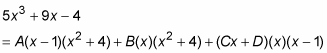4. Take the roots of the linear factors and plug them — one at a time — into x in the equation from Step 3, and then solve.

• If x = 0

–4 = –4A

A = 1

• If x = 1

10 = 5B

B = 2

You can’t solve for all the unknowns by plugging in the roots of the linear factors, so you have more work to do.

5. Plug into the Step 3 equation the known values of A and B and any two values for x not used in Step 4 (low numbers make the arithmetic easier) to get a system of two equations in C and D.

• A = 1 and B = 2, so

If x = –1

–18 = –10 – 10 – 2C + 2D

2 = –2C + 2D

1 = –C + D

• If x = 2

54 = 8 + 32 + 4C + 2D

14 = 4C + 2D

7 = 2C + D

6. Solve the system: 1 = –C + D and 7 = 2C + D.

You should get C = 2 and D = 3.

7. Split up the original integral and integrate.

Using the values obtained in Steps 4 and 6, A = 1, B = 2, C = 2, and D = 3, and the equation from Step 2, you can split up the original integral into three pieces: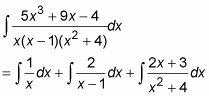And with basic algebra, you can split up the third integral above into two pieces, resulting in the final partial fraction decomposition: